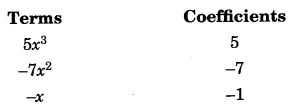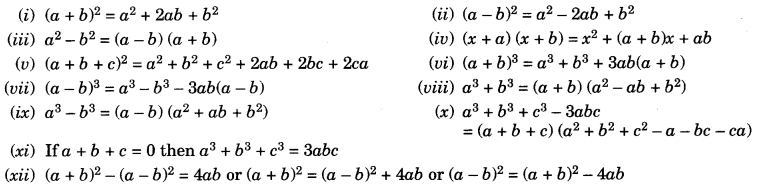On this page, you will find Polynomials Class 9 Notes Maths Chapter 2 Pdf free download. CBSE NCERT Class 9 Maths Notes Chapter 2 Polynomials will seemingly help them to revise the important concepts in less time.

## CBSE Class 9 Maths Chapter 2 Notes Polynomials

### Polynomials Class 9 Notes Understanding the Lesson

1. An expression which is the combination of constants and variables and are connected by some or all the operations addition, subtraction, multiplication and division is known as an algebraic expression.
Example: 7 + 9x – 2x2 + $$\frac{5}{6}$$ xy

2. Constant: Which has fixed numerical value.
Example: 7, -4, $$\frac{3}{4}$$ , n etc.

3. Variable: A symbol which has no fixed numerical value is known as a variable.
Example: 2x, 5x2

4. Terms: These are the parts of an algebraic expression which are separated by operations, like addition or subtraction are known as terms.
Example: In the expression 5x3 + 9x2 + 7x – 3, terms are 5x3, 9x2, 7x and -3

5. Polynomial: An algebraic expression of which variables have non-negative integral powers is called a polynomial.
Example:
(a) 5x2 + 7x + 3
(b) 9y3 – 7y2 + 3y + 7

6. Coefficient: A coefficient is the numerical value in a term.
Note: If a term has no coefficient, the coefficient is an unwritten 1.
Example: 5x3 – 7x2 – x + 37. Degree of a polynomial (in one variable): The highest power of the variable is called the degree of the polynomial.
Example: 5x + 4 is a polynomial in x of degree 1.

8. Degree of a polynomial in two or more variables: The highest sum of powers of variables is called the degree of the polynomial.
Example: 7x3 + 2x2y2 – 3ry + 8

9. Degree of polynomial = 4 (Sum of the powers of variables x and y )

10. Types of Polynomial

(i) Linear polynomial: A polynomial of degree one is called a linear polynomial.
Example: 2x + 3 is a linear polynomial in x.

(ii) Quadratic polynomial: A polynomial of degree 2 is called a quadratic polynomial.
Example: 5x2 – 7x + 4 is a quadratic polynomial.

(iii) Cubic polynomial: A polynomial of degree 3 is called a cubic polynomial.
Example: 3x3 + 7x2 – 4x + 9 is a cubic polynomial.

(iv) Biquadratic polynomial: A polynomial of degree 4 is called a biquadratic polynomial.
Example: 7x4 – 2x3 + 4x + 9 is a biquadratic polynomial.

11. Number of Terms in a Polynomial
Categories of the polynomial according to their terms:

(i) Moflomil A polynomial which has only one non-zero term is called a monomial.
Example: 7, 4x, $$\frac{4}{5}$$ xy, 7x2y3z5, all are monomials.

(ii) Binomial: A polynomial which has only two non-zero terms is called binomial.
Example: 2x + 7, 9x2 + 3, 3x2yz + 4x3y3z2, all are binomials.

(iii) Trinomial: A polynomial which has only three non-zero terms is called a trinomial.
Example: 5x2 + lx + 9, 5xy + 7xy2 + 3x3yz, all are trinomials.

(iv) Constant polynomial: A polynomial which has only one term and that is a constant is called a constant polynomial.
Example: $$\frac{-3}{4}$$, 7, 5 all are constant polynomials. 4
Note: The degree of constant non-zero polynomial is zero.

(v) Zero polynomial. A polynomial which has only one term i.e., 0 is called a zero polynomial.
Note: Degree of a zero polynomial is not defined.

12. Value of a Polynomial

Value of a polynomial is obtained, when variable of a given polynomial is interchanged or replaced by a ; constant.    Let p(x) is a polynomial then value of polynomial at x = a is p(a).
Zero or root of a polynomial: A zero or root of a polynomial is the value of that variable for which value of polynomial p(x) becomes zero i.e., p(x) = 0.
Let p(x) be the polynomial and x – a.
If p(a) = 0 then real value a is called zero of a polynomial.

13. Remainder Theorem
Let p(x) be a polynomial of degree ≥ 1 and a be any real number. If p(x) is divided by the linear polynomial x-a, then the remainder is p(a).

Proof: Let p(x) be any polynomial of degree greater than or equal to 1. When p(x) is divided by x – a, the quotient is q(x) and remainder is r(x).
i.e.,p(x) = (x-a) q(x) + r(x)
Since degree of x – a is 1 and the degree of r(x) is less than the degree of x – a so the degree of r(x) = 0.
It: means r(x) is a constant, say r.
Therefore, for every value of x,  r(x) = r
then   p(x) = (x-a) q(x) + r
When x = a, then  p(a) = (a – a) q(x) + r ⇒ p(a) = r

14. Factor Theorem
If p(x) is a polynomial of degree greater than or equal to 1 and a be any real number, then

• x – a is a factor of p(x) i.e., p(x) – (x-a) q(x) which shows x – a is a factor of p(x)
• Since x – a is a factor of p(x)
p(x) = (x-a)g(x) for same polynomial g(x). In this case,p(a) = (a-a) g (a) = 0

15. Factorisation of the Polynomial ax2 + bx + c by Splitting the Middle Term
Let           p(x) = ax2 + bx + c
and factor of polynomial p(x) = (px + q) and (rx + s)
then   ax2 + bx + c = (px + q) (rx + s) = prx2 + (ps +qr)x+ qs
Comparing the coefficient of x2 on both sides
a = pr …………. (1)
Comparing the coefficient of x
b =ps + qr …………. (2)
and comparing the constant terms
c = qs ……………..(3)
which shows that b is the sum of two numbers ps + qr.
Product of two numbers ps x qr =pr x qs = ac
So for factors ax2 + bx + c, we should write b as sum of two numbers whose product is ac.
Example: Factorise 6x2 + 17x + 5
Here,  b = p + q = 17
and   ac = 6 x 5 = 30 (= pq)
then we get factors of 30,      1 x 30, 2 x 15, 3 x 10, 5 x 6,
Among above factors of 30, the sum of 2 and 15 is 17
i.e.,p + q = 2 + 15 = 17
∴ 6x2 + 17x + 5 = 6x2 + (2 + 15)x + 5 = 6x2 + 2x + 15x + 5
= 2x(3x + 1) + 5(3x + 1) = (3x + 1) (2x + 5)

16. Algebraic Indentities